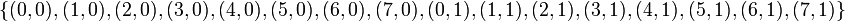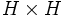# Diagonally embedded Z4 in direct product of Z8 and Z2

This article is about a particular subgroup in a group, up to equivalence of subgroups (i.e., an isomorphism of groups that induces the corresponding isomorphism of subgroups). The subgroup is (up to isomorphism) cyclic group:Z4 and the group is (up to isomorphism) direct product of Z8 and Z2 (see subgroup structure of direct product of Z8 and Z2).
The subgroup is a normal subgroup and the quotient group is isomorphic to cyclic group:Z4.
VIEW: Group-subgroup pairs with the same subgroup part | Group-subgroup pairs with the same group part| Group-subgroup pairs with the same quotient part | All pages on particular subgroups in groups

Here, the group$G$ is the group direct product of Z8 and Z2, written for convenience using ordered pairs with the first element an integer mod 8 (coming from cyclic group:Z8) and the second element an integer mod 2. The addition is coordinate-wise.$G$ has 16 elements:$\! \{ (0,0), (1,0), (2,0), (3,0), (4,0), (5,0), (6,0), (7,0), (0,1), (1,1), (2,1), (3,1), (4,1), (5,1), (6,1), (7,1) \}$

The subgroup$H$ is:$\! \{ (0,0), (2,1), (4,0), (6,1) \}$$H$ is isomorphic to cyclic group:Z4 and the quotient group$G/H$ is isomorphic to cyclic group:Z4 as well.

## Cosets$H$ has four cosets in$G$ (it is a normal subgroup since$G$ is an abelian group, so the left and right cosets coincide):$\! \{ (0,0), (2,1), (4,0), (6,1) \}, \{ (1,0), (3,1), (5,0), (7,1) \}, (2,0), (4,1), (6,0), (0,1) \}, \{ (3,0), (5,1), (7,0), (1,1) \}$

## Subgroup properties

### Invariance under automorphisms and endomorphisms: basic properties

Property Meaning Satisfied? Explanation
normal subgroup invariant under inner automorphisms Yes abelian implies every subgroup is normal
characteristic subgroup invariant under automorphisms Yes The elements$(2,1)$ and$(6,1)$ are the only elements of the group with the property that the square of the element has order two but the element is not itself a square. Any automorphism must therefore preserve or interchange these two elements, and hence must send$H$ (which is cyclic on both of them) to itself.
fully invariant subgroup invariant under all endomorphisms No The projection to the second direct factor (map$(a,b) \mapsto b$) does not preserve this subgroup. Nor does the projection to the first direct factor.
This is the smallest example for characteristic not implies fully invariant in finite abelian group. There are no analogous examples for odd order, because characteristic equals fully invariant in odd-order abelian group.

### Invariance under automorphisms and endomorphisms: advanced properties

Property Meaning Satisfied? Explanation
square-closed characteristic subgroup The square of$H$ (direct product$H \times H$) is characteristic in$G \times G$. No PLACEHOLDER FOR INFORMATION TO BE FILLED IN: [SHOW MORE]
finite direct power-closed characteristic subgroup Any finite direct power of$H$ is characteristic in the corresponding finite direct power of$G$. No Follows from not being square-closed characteristic.